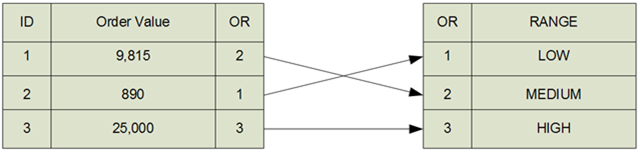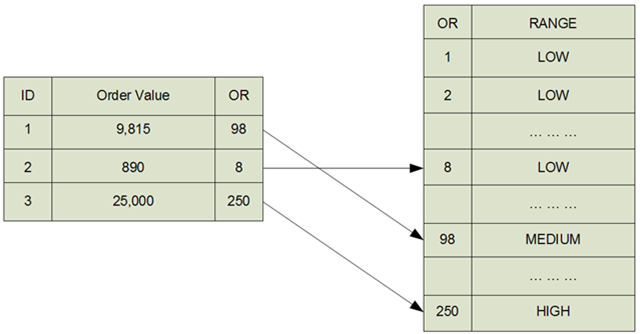A ranged dimension is a dimension that is used to have a discrete view of a continuous measure.

A good example of this is the analysis of amount sold per order. In AdventureWorks we have, for each line of an order, the amount and quantity sold. We would like to divide orders in three groups (HIGH, MEDIUM, LOW) based on the total of the order. This is a very frequent kind of analysis that is used to determine how much money customers are willing to spend in a single order and may be very useful to check whether there is any kind of relationship between the characteristics of a customer and his/her medium spending capability.

In SQL it is very easy to get this analysis:

`WITH     OrderTotals AS (  SELECT              SalesOrderId = SalesOrderId,              OrderValue   = SUM (OrderQty * UnitPrice)  FROM              Sales.SalesOrderDetail         GROUP BY             SalesOrderId),     OrdersRanged AS (  SELECT             SalesOrderId,             OrderValue,             OrderRange = CASE  WHEN OrderValue <= 1000  THEN 'LOW'  WHEN OrderValue <= 10000 THEN 'MEDIUM'  ELSE 'HIGH'  END  FROM             OrderTotals) SELECT     OrderRange  = OrderRange,      OrderNumber = COUNT (*),     OrderValue  = SUM (OrderValue) FROM     OrdersRanged GROUP BY     OrderRange  `

OrderRange OrderNumber OrderValueHIGH 1,827 75,745,964.3559MEDIUM 12,574 31,075,706.5634LOW 17,064 3,552,218.3941

This SQL query is correct but what we really want to do is to create a new OrderRange dimension in our BI solution to let the user further analyze the characteristics of the orders. The granularity of this attribute is at the Order level and so we have to add an ID_ValueRange column in the fact table of orders.

If we define the Dim OrderRange dimension we will get something like it:

• ID_OrderRange Primary Key, usually INT IDENTITY
• MinimunValue Left value for the range
• MaximunValue Right value for the range
• OrderRange Textual description of the range

At the end of the processing, our relational database will look like this:This solution is good when we have a very clear definition of what the ranges are during design time. This often does not happen in the real world. More likely, we will let the user define ranges through a table in the configuration database and we will define several attributes and hierarchies in the dimension to analyze how orders are ranged. All these attributes and hierarchies should be derived from the configuration database and processed during the ETL phase.

Ranges are dimensions that have a high variation rate over time and – normally – they can be defined only after the data is available because, before then, users do not have a clear understanding of what kind of ranges can be useful or not.

This leads to a very annoying problem i.e. the ranges can be determined only at the cube process time and not at ETL time. In other words we are not able to compute the ID_OrderRange key used to join the dimension to the fact table because the ranges change over time due to the user change of mind. Please note that in this case it is the dimension that changes its meaning, not the facts.

Computing the ranges during cube process time will lead us to define queries for the fact table that are complicated and uselessly consume processor time. So the challenge is to avoid this situation by means of changing the dimensional model.

The solution that we recommend is to define ranged dimensions with a different surrogate key. Even if we are not able to define the exact ranges, we can normally define the maximum granularity that will be used to define ranges. In the example the granularity can be 1,000, but it can be more cleverly set to 100 in order to gain some flexibility at the lowest level of the range. Supposing that 100,000 is the maximum value of an order (at least from the DSS analyst point of view), we will have a maximum range of values that starts at 100 and ends at 100,000 with a step of 100, leading to a range of only 1,000 different values. This will be the Dim OrderRange dimension.

Once we have defined the granularity we can redefine the Dim_OrderRange assigning to ID_OrderRange a simple INTEGER field that represents the value of FLOOR (OrderRange / 100). The same value, computed for each column of the order fact table, will be the ID used to join the fact table to the dimension.

The dimension will contain all the values from 0 to the maximum order value divided by the granularity and so it will be larger but still contain only a few thousand rows. In our example the table switched from 3 values to 1,000 but, from SSAS point of view, it is still a very small dimension. Moreover, as the distinct values of the attributes are still three, the aggregation will work very well leading to optimum performances.

The situation, with ranged dimensions, will be this:Using the ranged dimension pattern you will be able to change all the attributes of the ranged dimension without having to worry about changes. The fact table will remain valid unless you decide to change the granularity.

Granularity is not subject to changes because it can be normally set to a value clearly defined by the user and there is very rarely the need of updating it.

Clearly ranged dimensions are a variation of standard dimension because their surrogate key has a clear meaning while the surrogate keys, in Kimball’s methodology, should not have any meaning at all. Nevertheless, we believe that in specific situation (and ranged dimension are among those) the “no meaning at all” constraint can be relaxed in order to get maximum flexibility in the final solution the customer will use.

Another very common situation where ranged dimension are useful is in the ranging of time duration. When handling time you can easily set the granularity to days, months, years or seconds, depending on the minimum and maximum values that the duration will have. Once done it you can compute the duration using the correct granularity and define any ranged dimension on it.

SUMAdds all the numbers in a column.

`SUM ( <ColumnName> )`

COUNTCounts the number of rows in the table where the specified column has a non-blank value.

`COUNT ( <ColumnName> )`

INTRounds a number down to the nearest integer.

`INT ( <Number> )`

FLOORRounds a number down, toward zero, to the nearest multiple of significance.

`FLOOR ( <Number>, <Significance> )`